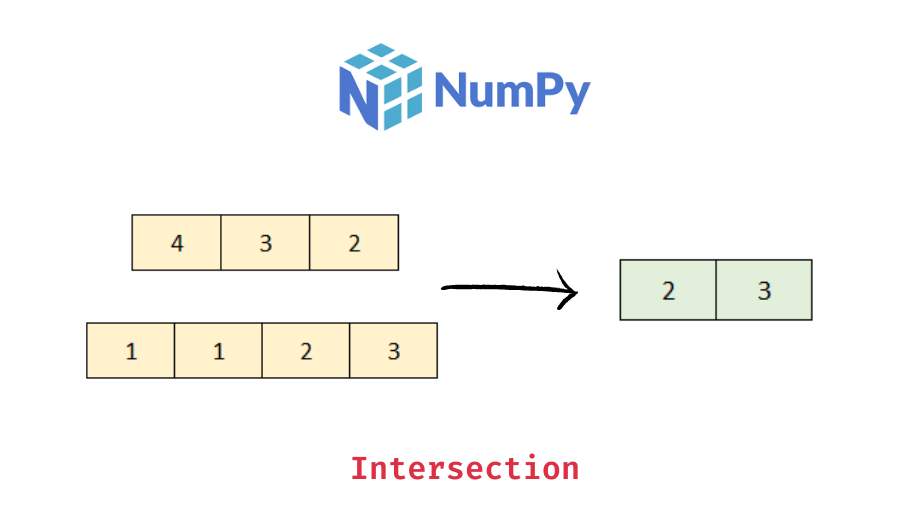# Python – Get Intersection of Two Numpy Arrays

In this tutorial, we will look at how to get the intersection of elements between two numpy arrays with the help of some examples.

You can use the numpy `intersect1d()` function to get the intersection (or common elements) between two numpy arrays. If the input arrays are not 1d, they will be flattened. The following is the syntax:

```import numpy as np
common_elements = np.intersect1d(ar1, ar2, assume_uniqe=False, return_indices=False)```

It returns the sorted, unique values that are present in both of the input arrays.

If the input arrays contain unique values, you can pass `True` to the `assume_uniqe` parameter, which can speed up the calculation. Note that, its default value is `False`, that is, it does not assume the input arrays to contain only unique values.

Pass `True` to the `return_indices` parameter if you also want the indices of the common values in the two arrays. It is `False` by default.

Let’s look at some examples –

Pass the two arrays as arguments to the numpy intersect1d() function.

```import numpy as np

# create two 1d arrays
ar1 = np.array([1, 2, 2, 1, 3])
ar2 = np.array([2, 3, 2, 3])
# intersection b/w the two arrays
common_elements = np.intersect1d(ar1, ar2)
# display the intersection array
print(common_elements)
# display the type
print(type(common_elements))```

Output:

📚 Data Science Programs By Skill Level

Introductory

Intermediate ⭐⭐⭐

🔎 Find Data Science Programs 👨‍💻 111,889 already enrolled

Disclaimer: Data Science Parichay is reader supported. When you purchase a course through a link on this site, we may earn a small commission at no additional cost to you. Earned commissions help support this website and its team of writers.

```[2 3]
<class 'numpy.ndarray'>```

You can see that the returned array has only the common elements between the two arrays.

You can use the same code as above or additionally pass `assume_unique=True` to speed up the computation (you wouldn’t get much difference for smaller arrays)

```# create two 1d arrays with unique values
ar1 = np.array([1, 2, 3])
ar2 = np.array([2, 3])
# intersection b/w the two arrays
common_elements = np.intersect1d(ar1, ar2, assume_unique=True)
# display the intersection array
print(common_elements)```

Output:

`[2 3]`

Pass `True` to the `return_indices` parameter and make sure you correctly collect the return values.

```# create two 1d arrays
ar1 = np.array([1, 2, 2, 1, 3])
ar2 = np.array([2, 3, 2, 3])
# intersection b/w the two arrays
common_elements, ar1_i, ar2_i = np.intersect1d(ar1, ar2, return_indices=True)
# display the intersection array
print(common_elements)
# display the indices
print(ar1_i)
print(ar2_i)```

Output:

```[2 3]
[1 4]
[0 1]```

You can see that we get the common values and their respective indices in both the input arrays.

If the input arrays are not 1d, they’ll be flattened and then the intersection will be computed.

```# create two 2d arrays
ar1 = np.array([[1, 1],
[2, 3]])
ar2 = np.array([[4, 5],
[3, 1]])
# intersection b/w the two arrays
common_elements = np.intersect1d(ar1, ar2)
# display the intersection array
print(common_elements)
# display the type
print(type(common_elements))```

Output:

```[1 3]
<class 'numpy.ndarray'>```

You can see that we get a 1d array with the common elements between both the input arrays as the return value.

For more on the numpy intersect1d() function, refer to its documentation.

With this, we come to the end of this tutorial. The code examples and results presented in this tutorial have been implemented in a Jupyter Notebook with a python (version 3.8.3) kernel having pandas version 1.0.5

•